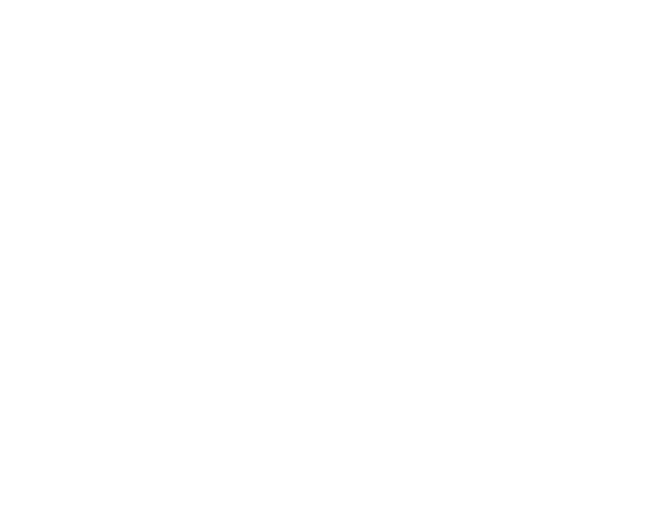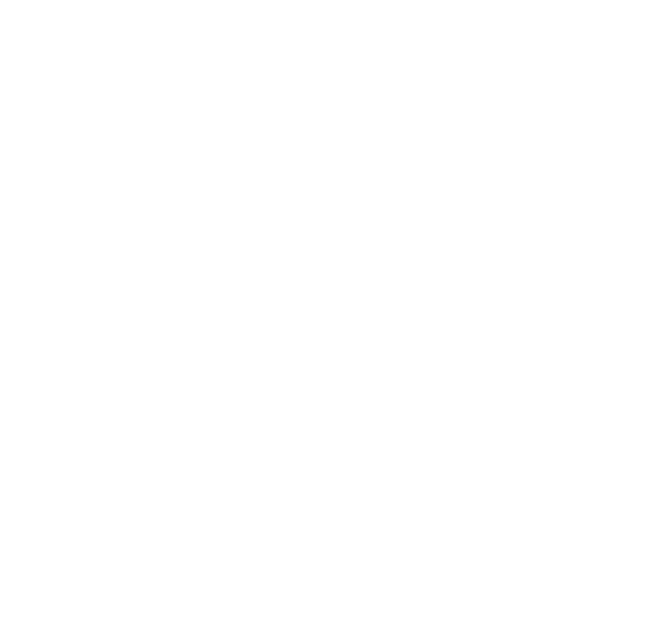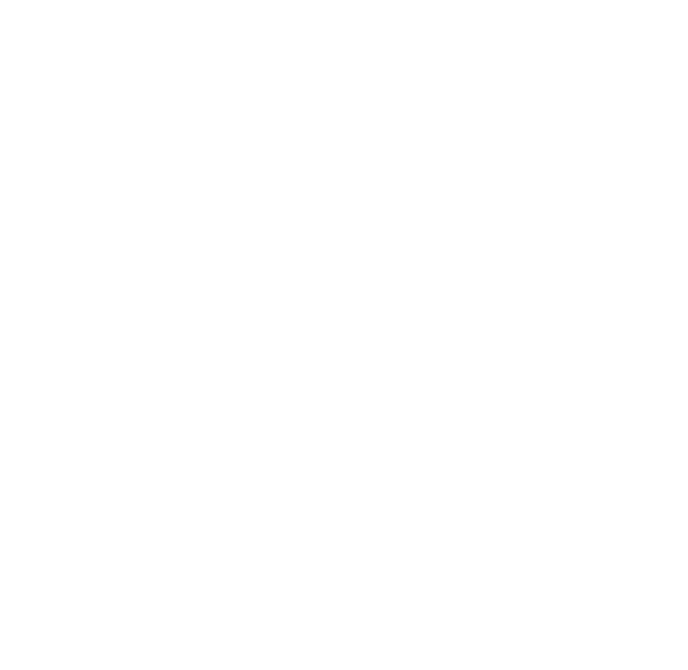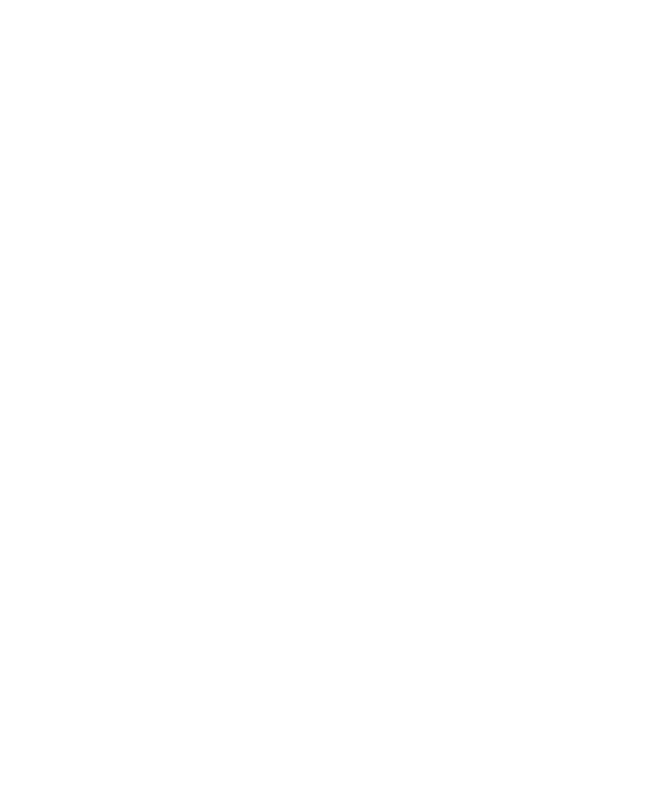# VBA Loop Through an Array

- by Puneet

Contents

In VBA, to loop through an array you can use the For Loop (For Next). And to write this loop code you need to know the size of the array (upper and lower bound) so that you can use both as the counter for the loop. Basically, for loop will start from the first element of the array and loop up to the last.

You have the following array with five elements from 1 to 5 and in these elements, you have numbers. So, the idea is to write a code that can loop through all these five elements and then multiply it with 2.

## Loop Through an Array in VBA

1. First, you need to know the upper and lower bounds of the array and for this, you need to use the UBOUND and LBOUND functions.2. After that, you need to use these bounds to create a counter for the loop.3. Next, you need to write a line code to multiply the element’s value with the 2. This line of code will take value from the element itself and then multiply it.4. In the end, use the “Next” keyword to close the loop code.Here’s the full code.

``````Option Base 1
Sub vba_array_loop()

Dim myArray(5) As Integer
myArray(1) = 10
myArray(2) = 20
myArray(3) = 30
myArray(4) = 40
myArray(5) = 50

Dim uB As Integer, lB As Integer
uB = UBound(myArray)
lB = LBound(myArray)

For i = lB To uB
myArray(i) = myArray(i) * 2
Next i

End Sub``````